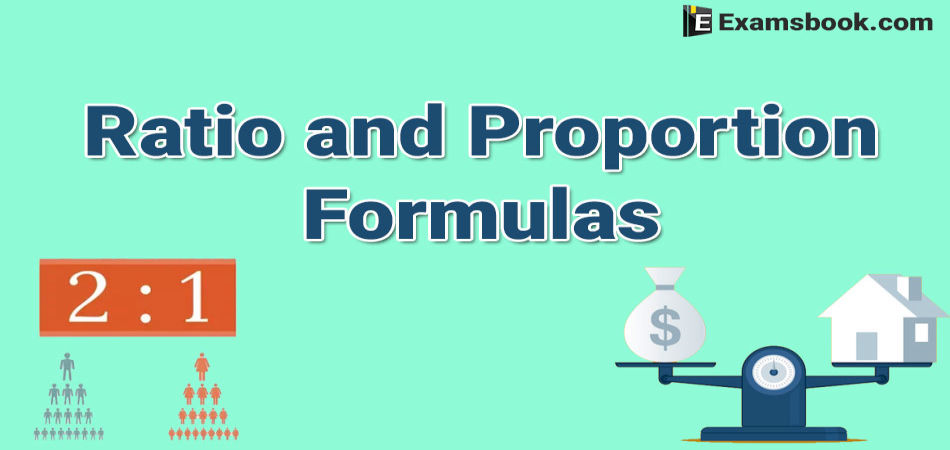• Save

Ratio and Proportion Formulas for Competitive Exams

10 months ago 2.5K ViewsRatio and Proportion questions take time to solve in the competitive exams. Students can solve easily these questions if they know ratio and proportion formulas that how to use formulas in these questions. So, here I am sharing ratio and proportion formulas to save your time in the examination.

You should practice with these formulas and should learn how to use formulas correctly while solving ratio and proportion questions. So, let's practice and improve your skill also to score high in the competitive exams.

Learn here in this blog, how to solve ratio and proportion problems with examples and different-2 equations.

Formulas for Ratio and Proportion Questions:

1. Ratio : The ratio of two quantities a and b in the same units, is the fraction a/b and we write it as a:b.

In the ratio a : b, we call a as the first term or antecedent and b, the second term or consequent.

Ex. The ratio 5 : 9 represent 5/9 with antecedent = 5, consequent = 9

Rule: The multiplication or division of each term of a ratio by the same non-zero number does not affect the ratio.

Ex. 4:5 = 8 : 10 = 12: 15 etc. Also, 4:6 = 2 : 3.

2. Proportion : The equality of two ratios is called proportion.

If a : b = c : d, we write, a : b : : c : d and we say that a, b, c, d are in proportion. Here a and d are called extremes, while b and c are called mean terms.

Product of means = Product of extremes.

Thus, a : b :: c : d ↔ (b×c) = (a×d).

3. (i) Fourth Proportional : If a : b = c : d, then d is called the fourth proportional to a, b, c.

(ii) Third Proportional : If a : b = b : c, then c is called the third proportional to a and b.

(iii) Mean Proportional : Mean Proportional between a and b is √ab.

4. (i) Comparison of Ratios :

We say that (a:b)>(c:d)↔ (a/b)>(c/d).

(ii) Compound Ratio :

The compound ratio of the ratios (a:b), (c:d), (e:f) is (ace: bdf).

5.(i) Duplicate ratio of (a:b) is (a2:b2).

(ii) Sub-duplicate ratio of (a:b) is (√a:√b).

(iii) Triplicate ratio of (a:b) is (a3 : b3).

(iv) Sub-triplicate ratio of (a:b) is (a1/3 : b1/3).

(v) If a/b = c/d, then (a+b)/(a-d) = (c+d)/(c-d).

6. Variation :

(i) We say that x is directly proportional to y, if x = ky for some constant k and we write, X α Y.

(ii) What say that x is inversely proportional to y, if xy = k for some constant k and we write, X α 1/y.

Example of Ratio and Proportion

Ex.1. If a : b = 5:9 and b : c = 4 : 7, find a : b : c.

Solution

a : b = 5 : 9 and b : c = 4 : 7 =[ 4×(9/4)] :[ 7×(9/4)] = 9 : (63/4).

→ a : b : c = 5 : 9 : (63/4)= 20 : 36 : 63.

Ex2. Find

(i) The fourth proportional to 4, 9,12.

(ii) The third proportional to 16 and 36;

(iii) The mean proportional between 0.08 and 0.1.8.

Solution

(i) Let the fourth proportional to 4, 9, 12 be X.

Then, 4 : 9 : : 12 : X ↔ 4 × X = 9 × 12 ↔ x = (9×12)/4 = 27.

Forth proportional to 4, 9, 12, is 27.

(ii) Let the third proportional to 16 and 36 be x.

Then, 16 : 36 : : 36: X  ↔ 16 × X = 36 × 36 ↔ X = (36×36)/16 =81.

Third Proportional to 16 and 36 is 81.

(iii) Mean proportional between 0.08 and 0.18

$$= \sqrt {0.08× 0.18 }= \sqrt{{8\over100}× {8\over100}}= \sqrt { {144\over100×100}} \ ={12\over100}= 0.12.$$

I hope these formulas are helpful for you. If you have any doubt or you want to ask amnything regarrding ratio and proportion formulas, you can ask me in the comment section.